# Concepts of Triangles

This set of MCQs helps you brush up on important math topics and prepare you to dive into skill practice.

Start Quiz

Name the postulate, if possible, that makes the triangles congruent.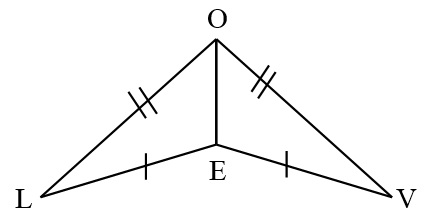ASA

SSS

SAS

Not possible

Name the corresponding angle or side.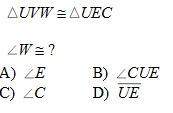A

B

C

D

Complete the congruence statement.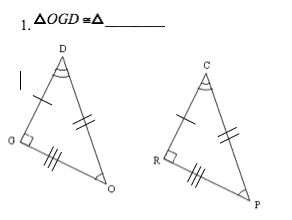CRP

PRC

PCR

RPC

Name the postulate, if possible, that makes the triangles congruent.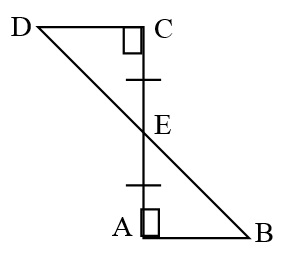SSS

SAS

ASA

Not possible

Two triangles are_______.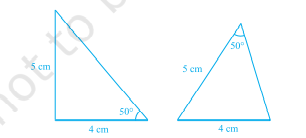Congruent

Non Congruent

D is the midpoint of the hypotenuse AC of a right △ABC. If AC=26 cm, then the length of BD is:

16

13

26

24

The length of PQPQ in the given figure is ___ cm.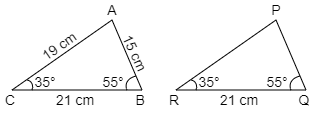25

12

15

14

If AD is the median of △ABC, then: